## Wednesday, November 5, 2014

### Pruning trees to one member per genus, and to one descendant for each clade younger than a particular age

The following request was recently posted to the R-sig-phylo email list:

“(1) I'd like to drop all but one of the tips in each genus (i.e. to generate a genus-level phylogeny). Given that all genera are monophyletic, it shouldn't matter which species I pick.

(2) I'd like to establish an era (e.g. 25 Mya) and leave only one representative of the clades there were present at that point in time.”

I submitted my replies (1, 2) but I'll repeat them here anyway.

First, let's simulate scenario (1) in which we have a species-level tree with one or more species per genus, and want to reduce the tree to a genus tree:

``````library(phytools)
## here are our species names
tips<-c("Abc_def","Abc_ghi","Def_ghi","Def_jkl",
"Ghi_jkl","Ghi_mno","Jkl_mno","Jkl_pqr")
tree<-stree(n=8,type="balanced",tip.label=tips)
plotTree(tree,ftype="i")
``````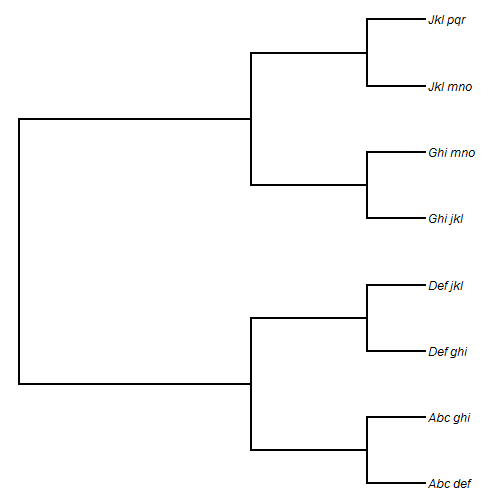Next, we'll get a list of all genus names, and prune the tree of all but one member of each genus:

``````## get a list of all genera
tips<-tree\$tip.label
genera<-unique(sapply(strsplit(tips,"_"),function(x) x))
## here are our genera
genera
``````
``````##  "Abc" "Def" "Ghi" "Jkl"
``````
``````## now drop all but one of each
ii<-sapply(genera,function(x,y) grep(x,y),y=tips)
tree<-drop.tip(tree,setdiff(tree\$tip.label,tips[ii]))
plotTree(tree,ftype="i")
``````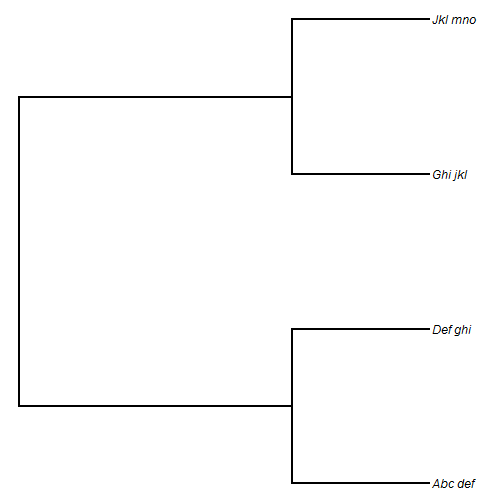Finally, we can rename our tips to be the genus names only:

``````tree\$tip.label<-sapply(strsplit(tree\$tip.label,"_"),function(x) x)
plotTree(tree,ftype="i")
``````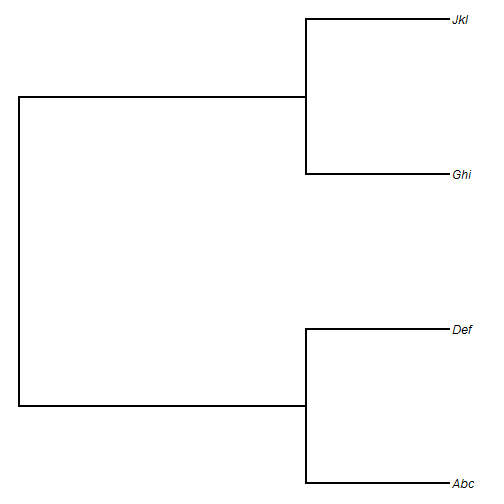That's it.

Now, let's move on to part (2). For this, I'll simulate a true tree of arbitrary total depth `100`.

``````tree<-pbtree(n=100,scale=100)
plotTree(tree,fsize=0.6)
``````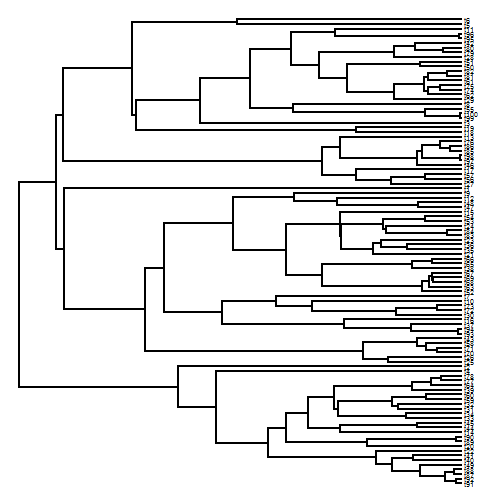Now, let's prune each clade younger than 25 units of time old to include only 1 descendant:

``````## get all node heights
H<-nodeHeights(tree)
## time from the root
t<-max(H)-25
## identify all edges that cross 25 mybp
h1<-which(H[,1]<t)
h2<-which(H[,2]>t)
ii<-intersect(h1,h2)
## all daughter nodes of those edges
nodes<-tree\$edge[ii,2]
## internal phytools function
getDescendants<-phytools:::getDescendants
## find all descendants from each edge
tips<-lapply(nodes,getDescendants,tree=tree)
## find all tips to keep
tips<-tree\$tip.label[sapply(tips,function(x,y) x[x<=Ntip(y)],y=tree)]
## drop all the others
tree<-drop.tip(tree,setdiff(tree\$tip.label,tips))
plotTree(tree)
lines(c(t,t),par()\$usr[3:4],lty="dashed",col="red")
``````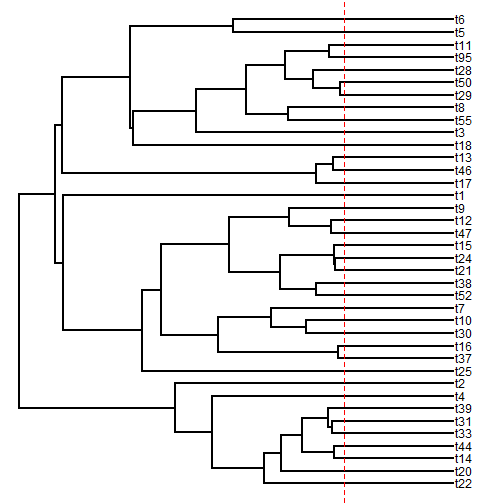Cool. It worked!

That's all for now.

1.Great post Liam, thanks!
These steps to prune trees are more or less the opposite of David Bapst's expandTaxonTree function from paleotree (in case anyone's wondering)

2.3.This comment has been removed by the author.

4.This comment has been removed by the author.

5.That's really helpful. Would it be possible to randomize the removal of species tips so one random tip was left to represent the genus?

Note: due to the very large amount of spam, all comments are now automatically submitted for moderation.